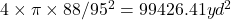A theme park has a ride that is located in a sphere. The ride goes around the widest circle of the sphere which has a circumference of 558.9

Question

A theme park has a ride that is located in a sphere. The ride goes around the widest circle of the sphere which has a circumference of 558.92 yd. What is the surface area of the sphere?

in progress 0
5 months 2021-09-02T15:04:37+00:00 1 Answers 7 views 0

The surface area of the sphere is =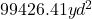Step-by-step explanation:

The area of a sphere can be calculated using this formula: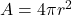However, we have a little problem: we do not have our radius yet to utilize in the formula above.

We can get our radius from the circumference of the circle which has been given to us.

circumference =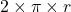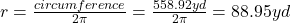We now have our radius. we can input it into equation 1 above

The Surface area of sphere =